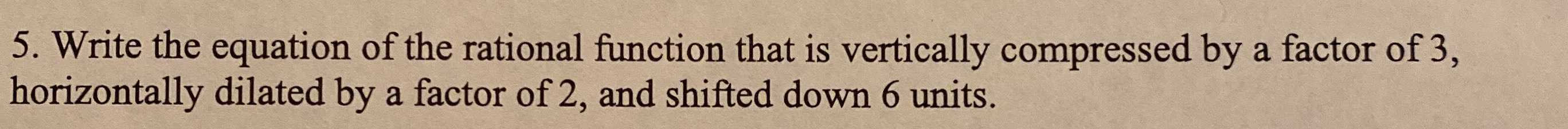### Still have math questions?

Algebra
Question5. Write the equation of the rational function that is vertically compressed by a factor of $$3$$ , horizontally dilated by a factor of $$2$$ , and shifted down $$6$$ units.